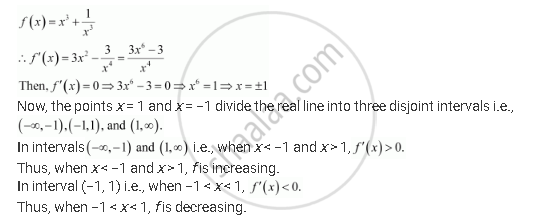Share

# Find the Intervals in Which the Function F Given by F(X) = X^3 + 1/X^3 X != 0, is - CBSE (Commerce) Class 12 - Mathematics

ConceptIncreasing and Decreasing Functions

#### Question

Find the intervals in which the function f given by f(x) = x^3 + 1/x^3 x != 0, is

(i) increasing

(ii) decreasing

#### SolutionIs there an error in this question or solution?

#### APPEARS IN

NCERT Solution for Mathematics Textbook for Class 12 (2018 to Current)
Chapter 6: Application of Derivatives
Q: 7 | Page no. 242

#### Video TutorialsVIEW ALL 

Solution Find the Intervals in Which the Function F Given by F(X) = X^3 + 1/X^3 X != 0, is Concept: Increasing and Decreasing Functions.
S